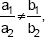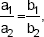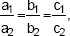Linear Equations in two variables-Notes

Class X Math
Notes for Pair of Linear Equations in Two Variables
Basic Concepts with Examples
•    Linear Equation in Two Variables
An equation which can be put in the form
ax + by + c = 0
where a, b and c are real numbers {a, b ≠ 0) is called a linear equation in two variables ‘x’ and ‘y’
•    General Form of a Pair of Linear Equations in Two Variables
General form of a linear pair of equations in two variables is:
a1x + b1y + cx - 0 and
a2x + b2y + c2 = 0
where a1 b1, c1, a2, b2, c2 are real numbers such that
a12 + b12 0 and a22 + b22 0
•    Solution of a Pair of Linear Equations in Two Variables
The solution of a linear equation in two variables ‘x’ and ‘y’ is a pair of values (one for ‘x’ and other for ‘y’)
which makes the two sides of the equation equal.
There are two methods to solve a pair of linear equations:
(i) algebraic method
(ii) graphical method.
•    Algebraic Method
We have already studied (i) Substitution method and (ii) Elimination method. Here, we will study cross-multiplication method also.
If a1 x + b1 y + c1 = 0
a2 x + b2 y + c2 = 0
form a pair of linear equations, then the following three situations can arise:
(i) Ifthen the system is consistent.
(ii) Ifthen the system is inconsistent.
(ii) Ifthen the system is dependent and consistent.
•    Graphical Method of Solution of a Pair of Linear Equations
(i) If the graphs of two equations of a system intersect at a point, the system is said to have a unique solution, i.e., the system is consistent.
(ii) If the graphs of two equations of a system are two parallel lines, the system is said to have no solution, i.e., the system is inconsistent.
(iii) When the graphs of two equations of a system are two coincident lines, the system is said to have infinitely many solutions, i.e., the system is consistent and dependent.